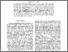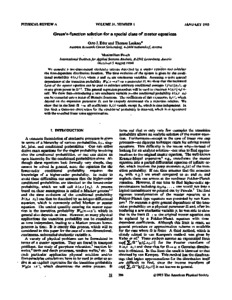# Green’s-function solution for a special class of master equations

Eder, O.J., Lackner, T., & (1985). Green’s-function solution for a special class of master equations. Physical Review A 31 (1) 366-377. 10.1103/PhysRevA.31.366.Preview
Text
Green’s-function solution for a special class of master equations.pdf - Published Version

## Abstract

We consider a one-dimensional stochastic process described by a master equation and calculate the time-dependent distribution function. The time evolution of the system is given by the conditional probability h(x,t‖x0), where x and x0 are continuous variables. Assuming a quite general dependence of the transition probability WΩ(x→x’) on a parameter Ω, we show that the backward form of the master equation can be used to calculate arbitrary conditional averages 〈f(x)‖x0⟩t up to any given power in Ω−1. This general expansion procedure will be used to construct h(x,t‖x0) itself. We show that—introducing a new stochastic variable y—the conditional probability h(x,t‖x0) can be expanded into a series of Hermite functions. The coefficients of this expansion, bn(t), which depend on the expansion parameter Ω, can be uniquely determined via a recursion relation. We show that in the limit Ω→∞ all coefficients bn(t) vanish, except b0, which is time independent. In this limit a Gaussian distribution for the conditional probability is obtained, which is in agreement with the so-called linear noise approximation.

Item Type: Article Acid Rain Program (ACI) Romeo Molina 15 Apr 2016 11:33 27 Aug 2021 17:26 http://pure.iiasa.ac.at/12722View Item